# 通过 EMR Notebooks 在运行的集群上安装 Python 库

Original URL:https://aws.amazon.com/blogs/big-data/install-python-libraries-on-a-running-cluster-with-emr-notebooks/

## 在EMR Notebooks中使用笔记本范围的Python库的优势

• 运行时安装 – 您可以从 PyPI 存储库导入您需要的 Python 库，并在需要时立即将它们安装到远程集群上。这些库可立即用于 Spark 运行时环境，无需重新启动笔记本会话或重新创建集群。
• 隔离依赖关系 – 使用 EMR Notebooks 安装的库是笔记本会话范围隔离的，不会干扰集群引导操作安装的库或从其他笔记本会话安装的库。这些笔记本范围的库会优先于引导操作的库被集群使用。多个笔记本用户可以各自导入和使用不同版本的库，而不会在同一集群上发生依赖关系冲突。
• 便携的库环境 – 库的安装将在您的笔记本文件中进行。这样一来，当您将笔记本切换到另一个集群时，就可以通过重新执行笔记本代码来重新创建库环境。当笔记本会话结束时，通过 EMR Notebooks 安装的库将自动从托管 EMR 集群中删除。

## 使用笔记本范围的库

``print("Welcome to my EMR Notebook!")``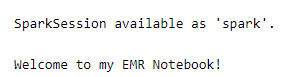``%%info``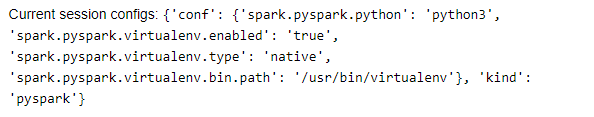``````%%configure -f
{ "conf":{
"spark.pyspark.python": "python",
"spark.pyspark.virtualenv.enabled": "true",
"spark.pyspark.virtualenv.type":"native",
"spark.pyspark.virtualenv.bin.path":"/usr/bin/virtualenv"
}
}``````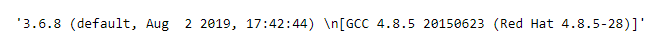``sc.list_packages()``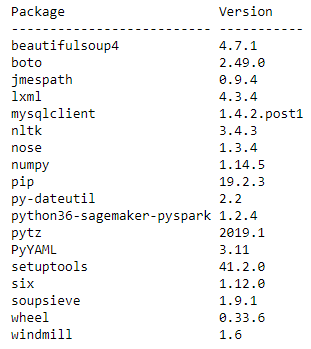``df = spark.read.parquet('s3://amazon-reviews-pds/parquet/product_category=Books/*.parquet')``

``````# Total columns
print(f'Total Columns: {len(df.dtypes)}')
df.printSchema()``````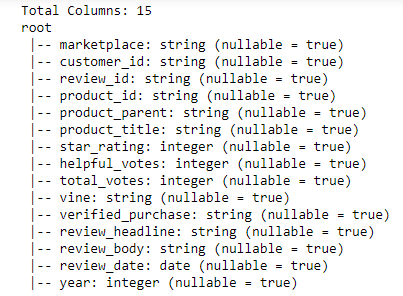``````# Total row
print(f'Total Rows: {df.count():,}')``````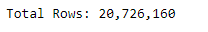``````# Total number of books
num_of_books = df.select('product_id').distinct().count()
print(f'Number of Books: {num_of_books:,}')``````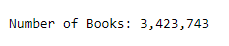``````sc.install_pypi_package("pandas==0.25.1") #Install pandas version 0.25.1
sc.install_pypi_package("matplotlib", "https://pypi.org/simple") #Install matplotlib from given PyPI repository``````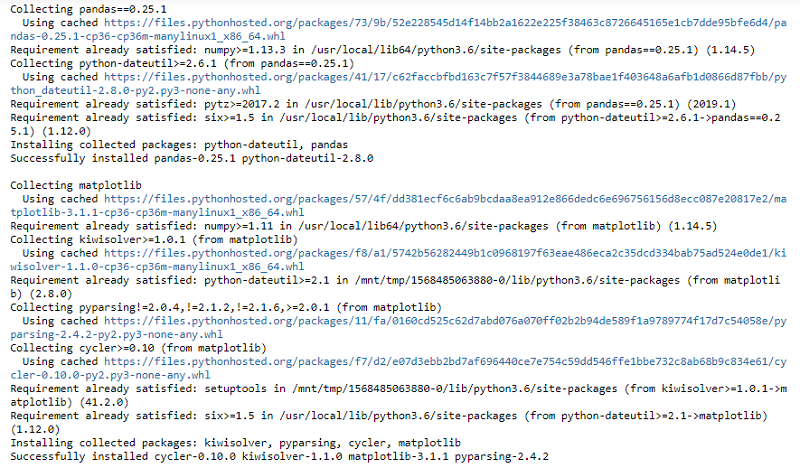PySpark API 中的install_pypi_package 将安装库以及任何关联的依赖。默认情况下，它会安装与您正在使用的 Python 版本兼容的最新版本的库。您还可以参考上面的Pandas 例子通过指定库版本来安装特定版本的库。

``sc.list_packages()``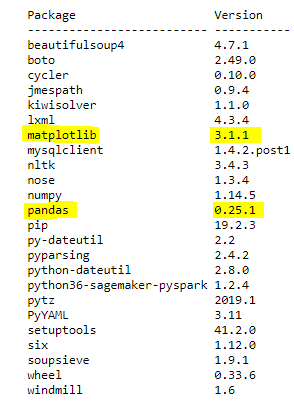``````# Number of reviews across years
num_of_reviews_by_year = df.groupBy('year').count().orderBy('year').toPandas()

import matplotlib.pyplot as plt
plt.clf()
num_of_reviews_by_year.plot(kind='area', x='year',y='count', rot=70, color='#bc5090', legend=None, figsize=(8,6))
plt.xticks(num_of_reviews_by_year.year)
plt.xlim(1995, 2015)
plt.title('Number of reviews across years')
plt.xlabel('Year')
plt.ylabel('Number of Reviews')``````

``%matplot plt``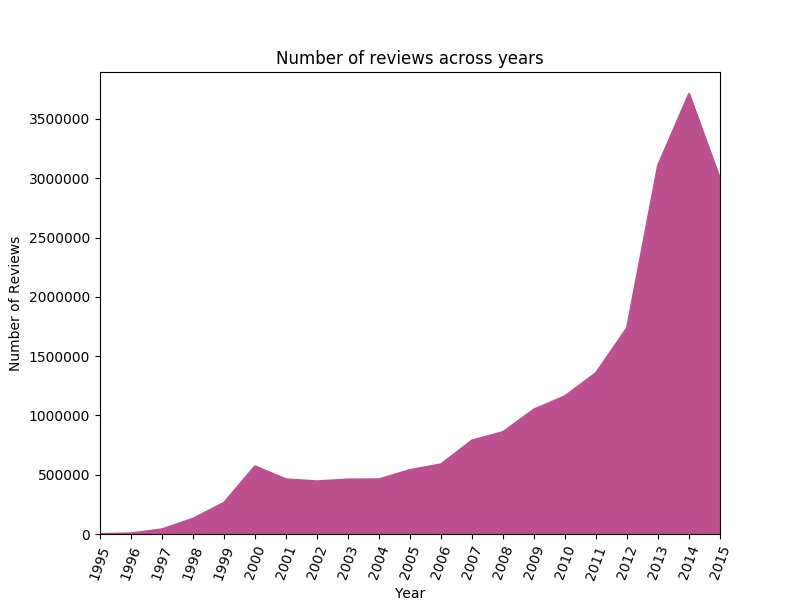``````# Distribution of overall star ratings
product_ratings_dist = df.groupBy('star_rating').count().orderBy('count').toPandas()

plt.clf()
labels = [f"Star Rating: {rating}" for rating in product_ratings_dist['star_rating']]
reviews = [num_reviews for num_reviews in product_ratings_dist['count']]
colors = ['#00876c', '#89c079', '#fff392', '#fc9e5a', '#de425b']
fig, ax = plt.subplots(figsize=(8,5))
w,a,b = ax.pie(reviews, autopct='%1.1f%%', colors=colors)
plt.title('Distribution of star ratings for books')
ax.legend(w, labels, title="Star Ratings", loc="center left", bbox_to_anchor=(1, 0, 0.5, 1))
``````

``%matplot plt``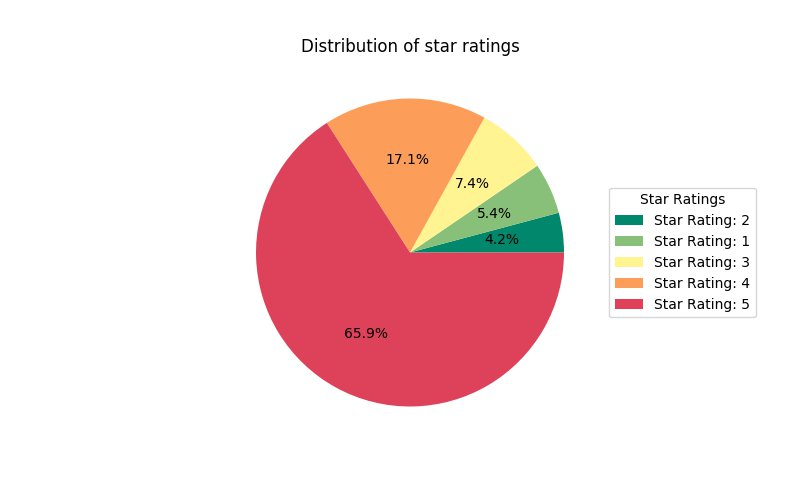``sc.uninstall_package('pandas')``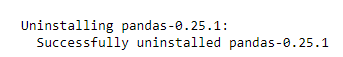``sc.list_packages()``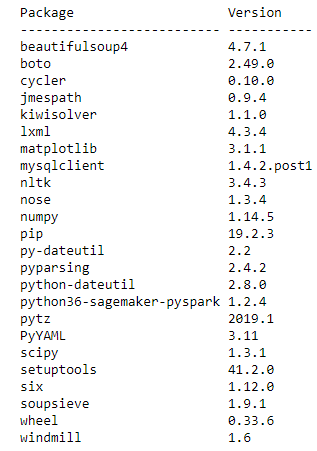## 在 EMR Notebooks 中使用本地 Python 库

``````%%local
conda list``````

``````kids_books = (
df
.where("lower(review_body) LIKE '%child%' OR lower(review_body) LIKE '%kid%' OR lower(review_body) LIKE '%infant%'OR lower(review_body) LIKE '%Baby%'")
.select("customer_id", "product_id", "star_rating", "product_title", "year")
)
``````

``````top_10_book_titles = kids_books.groupBy('product_title') \
.count().orderBy('count', ascending=False) \
.limit(10)
top_10_book_titles.show(10, False)``````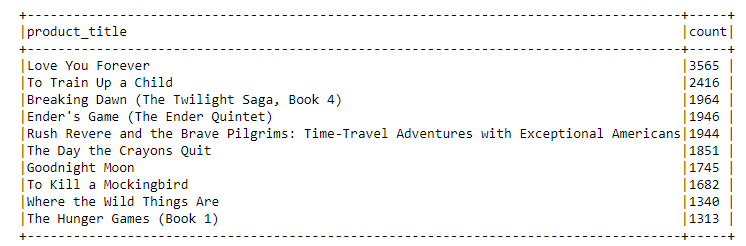``````top_10 = kids_books.groupBy('product_title', 'star_rating') \
.count().join(top_10_book_titles, ['product_title'], 'leftsemi') \
.orderBy('count', ascending=False)
top_10.show(truncate=False)``````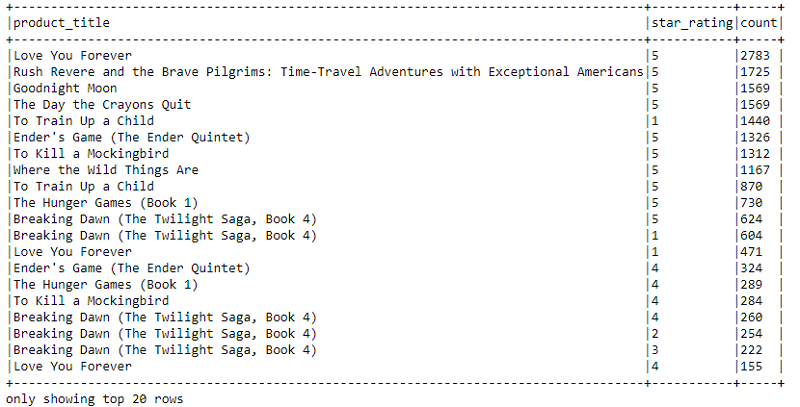``top_10.createOrReplaceTempView("top_10_kids_books")``

``````%%sql -o top_10 -n -1
SELECT product_title, star_rating, count from top_10_kids_books
GROUP BY product_title, star_rating, count
ORDER BY count Desc``````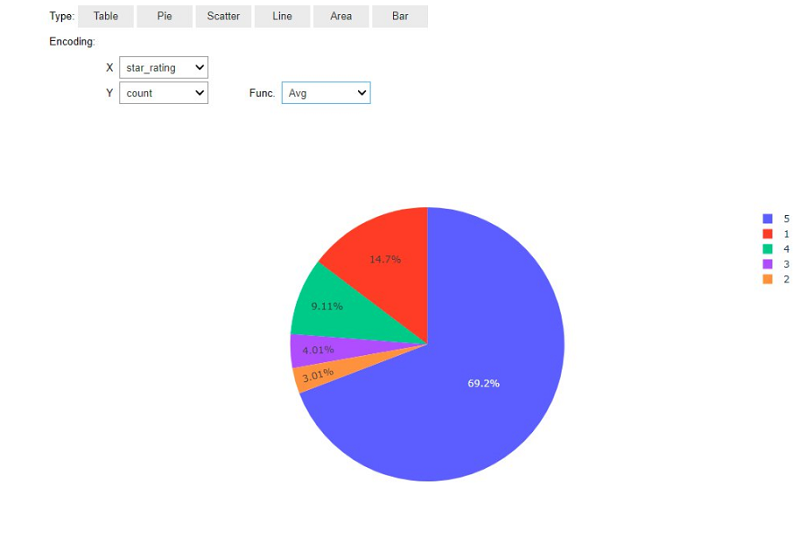``````%%local
%matplotlib inline
import matplotlib.pyplot as plt
import seaborn as sns
import pandas as pd
sns.set()
plt.clf()
top_10['book_name'] = top_10['product_title'].str.slice(0,30)
colormap = sns.color_palette("hls", 8)
pivot_df = top_10.pivot(index= 'book_name', columns='star_rating', values='count')
pivot_df.plot.barh(stacked=True, color = colormap, figsize=(15,11))
plt.title('Top 10 children books',fontsize=16)
plt.xlabel('Number of reviews',fontsize=14)
plt.ylabel('Book',fontsize=14)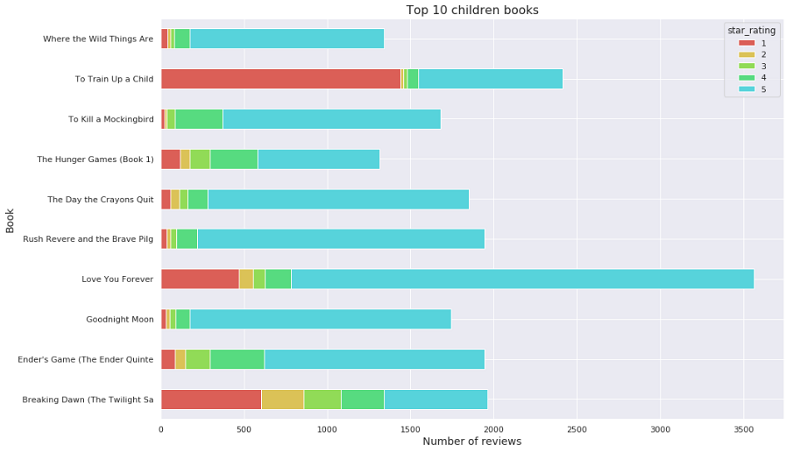## 小结

### 关于作者Parag Chaudhari 是 AWS 的一名软件开发工程师。

TAGS: